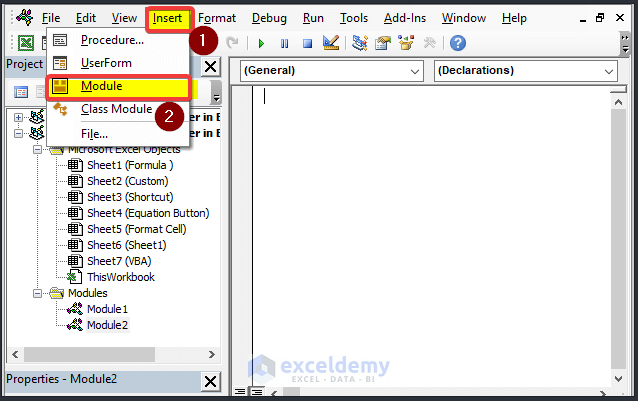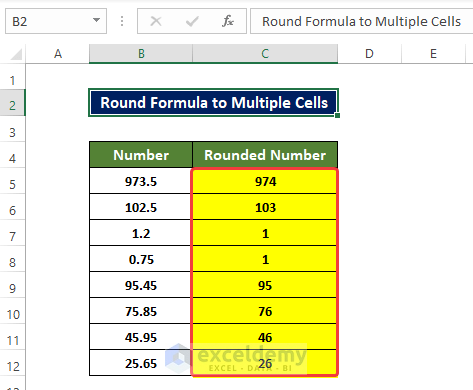# How to Add ROUND Formula to Multiple Cells in Excel (2 Easy Ways)

Get FREE Advanced Excel Exercises with Solutions!

We use numbers in Excel in different cases. In those cases, we may get numbers in long decimals. But in most real cases, those long decimals are not that significant. We often curtail those numbers to make those numbers round to the whole number, To become more accessible and easier to understand. Furthermore, we want to apply this rounding to all of the cells in a worksheet. In this article, we will discuss how you can add the ROUND formula to multiple cells in Excel.

## 2 Easy Ways to Add ROUND Formula to Multiple Cells in Excel

For demonstration purposes, we are going to use the below dataset. On the left-most Number column, we got the numbers with decimal points. And on the Rounded Number column, we got numbers already rounded up. How we apply the ROUND formula to all of the cells will discuss here.### 1. Dragging Fill Handle Icon to Apply ROUND Formula

After using the ROUND function, dragging the Fill Handle icon is the most common way to apply a formula to multiple cells in a range.

Steps

• In the beginning, select the cell D5 and enter the following formula:
`=ROUND(B5,0)`

After entering the formula you will notice that the cell now contains the rounded version of the number in cell B5.• Now in order to apply the ROUND formula to all cells, you need to drag the Fill Handle in the corner of cell D5 to cell D12. As shown in the image below.• After dragging the Fill Handle to cell D12, you will notice that the range of cells D5:D12 now contains the rounded version of the numbers in the range of cells B5:B12.Therefore, you can apply the ROUND formula to multiple cells in Excel by dragging the Fill Handle sign.

Read More: Round off Formula in Excel Invoice (9 Quick Methods)

### 2. Embedding VBA Macro to Round Multiple Cells in Excel

Applying a small macro can drastically reduce the hassle and time required for rounding multiple cells at a time.

Steps

• First, go to the Developer tab, then click Visual Basic.• Then click Insert > Module

##• In the Module window, enter the following code,
``````Sub Mul_round_formula()

Dim x As Range
Dim y As Range
Dim z As Integer

On Error Resume Next
xTitleId = "Enter Range of the Cells to be Rounded"

Set y = Application.Selection
Set y = Application.InputBox("Range", xTitleId, y.Address, Type:=8)
z = Application.InputBox("Decimal", xTitleId, Type:=1)

For Each x In y
x.Value = Application.WorksheetFunction.Round(x.Value, z)
Next

End Sub``````• Then close the window.
• After that, go to the View tab > Macros > View Macros.• After clicking View Macros, select the macros that you created just now. The name here is Mul_round_formula. Then click Run.• After clicking Run, a small new window will spawn.
• In that window, you need to just input the range of cells that you want to round.
• Select the range of cells, in this case it is \$C\$6:\$C\$12.
• Click OK after this.• After that, you will see then the range of cells C5:C12 is now rounded to the nearest integer.

##Therefore, you can apply the ROUND formula to multiple cells in Excel by using the VBA macro.

## How to Round Multiple Cells without ROUND Formula in Excel

Although you can round numbers in Excel using various formulas, remembering them and their syntaxis is quite a hassle. To get rid of that problem, we provided a solution below to round numbers in Excel without using the ROUND formula. This process will use the formatting option in the worksheet.

Steps

• In the beginning copy the values from the range of cells B5:B12 to the D5:D12.• Then select the values and right-click on the mouse.
• Next, from the context menu, click on the Format Cells options.• Then in the newly opened window, click on the Number tab, then click on the Number category.
• Set the Decimal places to 0.
• A preview of how the number will look will be in the Sample field above.
• Click OK after this.• After this, you will notice that the values in the range of C5:C12 are now rounded without any usage of formulas.## Conclusion

To sum it up, the question “how to add ROUND formula to multiple cells in Excel” is answered here by using two simple methods. We expand our discussion to protecting multiple rows and columns. Furthermore, we also discussed how we can protect active rows and columns in Excel. All of these methods require prior Excel-related knowledge to understand from scratch.

For this problem, a macro-enabled workbook is attached where you can practice these methods.

Feel free to ask any questions or feedback through the comment section. Any suggestion for the betterment of the Exceldemy community will be highly appreciable

## Related ArticlesRubayed Razib Suprov

Hi, I am Rubayed Razib Suprov. Graduated from the Bangladesh University of Engineering Technology in Naval Architecture and Marine Engineering department. I joined Exceldemy on March 2022. I have a keen interest in both fluid dynamics and data analysis. I try to teach people what I learned so far about the Excel tool to date, and hope I can make some positive impacts.

We will be happy to hear your thoughtsAdvanced Excel Exercises with Solutions PDF